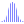#Covariance (PQL)

Returns the statistical covariance across two lists of numbers.

• Returned Output: Numeric
• Library: PQL \ Common \ Statistical
• Version: 2018.00.000
• Compatibility:
• Can be combined with and other PQL function throughout the application.
• It CANNOT be used with MDX or VBA functions. But it can be used on MDX-based content in other parts of the application.

#### Syntax

Covariance(, )

or

Covariance(, )

* Click on the function's arguments above for more details on the input values.

• The two lists of numbers can be typed in as a comma delimited list or inserted via an array.
##### Different Function types
• This function is like the Excel COVAR function - calculating a covariance across a 2 list of items.
• If you wish to find the covariance for the elements in a specified SET or LIST from a hierarchy, use this semantic function.

#### Examples

This example finds the covariance between 2 sets of numbers derived from columns 0 and 2.

Covariance(dataSetColumn(0), dataSetColumn(2))

Using the grid below, this would return the covariance value of -114.57